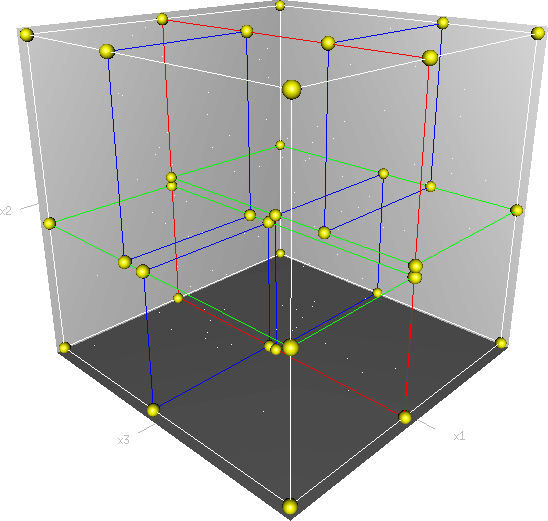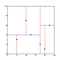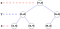# K Dimension TreeA 3D KD Tree model rendered which cuts the plane into two subcells

# What is a K-D Tree ?

A K-D Tree(also called as K-Dimensional Tree) is a binary search tree where data in each node is a K-Dimensional point in space. In short, it is a space partitioning data structure for organising points in a K-Dimensional space.

A non-leaf node in K-D tree divides the space into two parts, called as half-spaces.

Points to the left of this space are represented by the left subtree of that node and points to the right of the space are represented by the right subtree. We will soon be explaining the concept on how the space is divided and tree is formed.

# Understanding K-D Tree with an example

Let us number the planes as 0, 1, 2, …(K — 1). From the above example, it is quite clear that a point (node) at depth D will have A aligned plane where A is calculated as:

A = D mod K

How to determine if a point will lie in the left subtree or in right subtree?

If the root node is aligned in planeA, then the left subtree will contain all points whose coordinates in that plane are smaller than that of root node. Similarly, the right subtree will contain all points whose coordinates in that plane are greater-equal to that of root node.

Creation of a 2-D Tree:
Consider following points in a 2-D plane:
(3, 6), (17, 15), (13, 15), (6, 12), (9, 1), (2, 7), (10, 19)

1. Insert (3, 6): Since tree is empty, make it the root node.
2. Insert (17, 15): Compare it with root node point. Since root node is X-aligned, the X-coordinate value will be compared to determine if it lies in the rightsubtree or in the right subtree. This point will be Y-aligned.
3. Insert (13, 15): X-value of this point is greater than X-value of point in root node. So, this will lie in the right subtree of (3, 6). Again Compare Y-value of this point with the Y-value of point (17, 15) (Why?). Since, they are equal, this point will lie in the right subtree of (17, 15). This point will be X-aligned.
4. Insert (6, 12): X-value of this point is greater than X-value of point in root node. So, this will lie in the right subtree of (3, 6). Again Compare Y-value of this point with the Y-value of point (17, 15) (Why?). Since, 12 < 15, this point will lie in the left subtree of (17, 15). This point will be X-aligned.
5. Insert (9, 1):Similarly, this point will lie in the right of (6, 12).
6. Insert (2, 7):Similarly, this point will lie in the left of (3, 6).
7. Insert (10, 19): Similarly, this point will lie in the left of (13, 15)

How is space partitioned?
All 7 points will be plotted in the X-Y plane as follows:

Point (3, 6) will divide the space into two parts: Draw line X = 3.

Point (2, 7) will divide the space to the left of line X = 3 into two parts. horizontally.
Draw line Y = 7 to the left line X = 3.

Point (17, 15) will divide the space to the right of line X = 3 into two parts horizontally.
Draw line Y = 15 to the right of line X = 3.

Point (6, 12) will divide the space below line Y = 15 and to the right of line X = 3 into two parts.
Draw line X = 6 to the right of line X = 3 and below line Y = 15.

Point (13, 15) will divide the space below line Y = 15 and to the right of line X = 6 into two parts.
Draw line X = 13 to the right of line X = 6 and below line Y = 15.

Point (9, 1) will divide the space between lines X = 3, X = 6 and Y = 15 into two parts.
Draw line Y = 1 between lines X = 3 and X = 6.

Point (10, 19) will divide the space to the right of line X = 3 and above line Y = 15 into two parts.
Draw line Y = 19 to the right of line X = 3 and above line Y = 15.

# Implementation in Python Language

from collections import namedtuplefrom operator import itemgetterfrom pprint import pformatclass Node(namedtuple("Node", "location left_child right_child")):    def __repr__(self):        return pformat(tuple(self))def kdtree(point_list, depth: int = 0):    if not point_list:        return None    k = len(point_list)  # assumes all points have the same dimension    # Select axis based on depth so that axis cycles through all valid values    axis = depth % k    # Sort point list by axis and choose median as pivot element    point_list.sort(key=itemgetter(axis))    median = len(point_list) // 2    # Create node and construct subtrees    return Node(        location=point_list[median],        left_child=kdtree(point_list[:median], depth + 1),        right_child=kdtree(point_list[median + 1 :], depth + 1),    )def main():    """Example usage"""    point_list = [(7, 2), (5, 4), (9, 6), (4, 7), (8, 1), (2, 3)]    tree = kdtree(point_list)    print(tree)if __name__ == "__main__":    main()

Output :This is the generated K-D TreePictorial representation of the K-D Tree

Student , Explorer , Sports Enthusiast

## More from Abhishek Negi

Student , Explorer , Sports Enthusiast